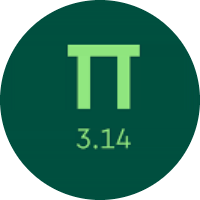# Understanding the Units of Measurement

In this quiz, you will learn about different units of measurement. You will learn about comparing and converting the customary units of length, weight, volume, etc. You will also get to know about the metric units.

Start Quiz12 tons

12 pounds15 milliliters

15 liters5 cups

1 quart

neither; they are equal

Convert.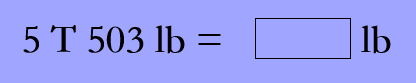5503

10503

21503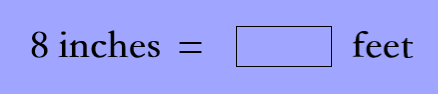1/3

1/2

2/37 kilometers

6,998 meters

neither; they are equal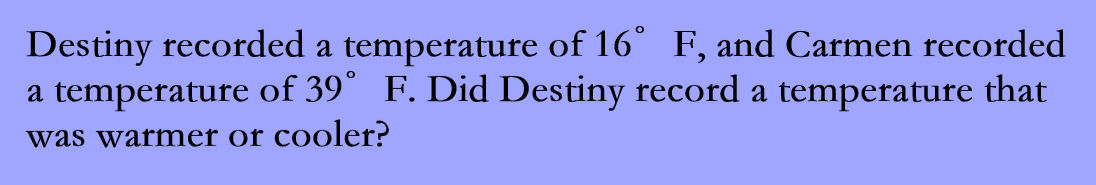warmer

cooler

Convert the temperature from degrees Celsius to degrees Fahrenheit, using the formula below.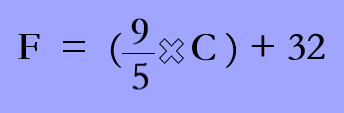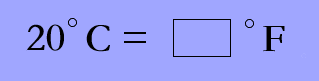68

48

58

Convert.80

800

8000

Quiz/Test Summary
Title: Understanding the Units of Measurement
Questions: 9
Contributed by: